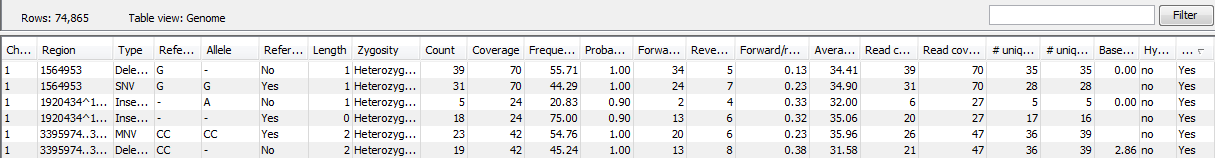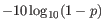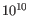## The variant track output

The variant track contains information on each of the variants called, including reference alleles. When opened in the table view there is a number of columns for each of the variants (see figure 22.86).Figure 22.86: A variant track shown in the table view.

The contents of these are:

Chromosome
The name of the reference sequence on which the variant is located.
Region
The region on the reference sequence at which the variant is located. The region may be either a 'single position', a 'region' or a 'between position region'.
Type
The type of variant. This can either be SNV (single-nucleotide variant), MNV (multi-nucleotide variant), insertion, deletion, or replacement. Learn more in Variant types.
Reference
The reference sequence at the position of the variant.
Allele
The allele sequence of the variant.
Reference allele
Describes whether the variant is identical to the reference. This will be the case for one of the alleles for most, but not all, detected heterozygous variants (e.g. the variant caller might detect two variants, A and G, at a given position in which the reference is 'A'. In this case the variant corresponding to allele 'A' will have 'Yes' in the 'reference allele' column entry, and the variant corresponding to allele 'G' would have 'No'. Had the variant caller called the two variants 'C' and 'G' at the position, both would have had 'No' in the 'Reference allele' column).
Length
The length of the variant. The length is 1 for SNVs, and for MNVs it is the number of allele or reference bases (which will always be the same). For deletions, it is the length of the deleted sequence, and for insertions it is the length of the inserted sequence. For replacements, both the length of the replaced reference sequence and the length of the inserted sequence are considered, and the longest of those two is reported.
Zygosity
The zygosity of the variant called, as determined by the variant caller. This will be either 'Homozygous', where there is only one variant called at that position or 'Heterozygous' where more than one variant was called at that position.
Count
The number of 'countable' fragments supporting the allele. The 'countable' fragments are those that are used by the variant caller when calling the variant. Which fragments are 'countable' depends on the user settings when the variant calling is performed - if e.g. the user has chosen 'Ignore broken pairs', reads belonging to broken pairs are not 'countable'. Note that, although overlapping paired reads have two reads in their overlap region, they only represent one fragment, and are counted only as one. (Please see the column 'Read count' below for a column that reports the value for 'reads' rather than for 'fragments').
Coverage
The fragment coverage at this position. Only 'countable' fragments are considered (see under 'Count' above for an explanation of 'countable' fragments). Note that, although overlapping paired reads have two reads in their overlap region, they only represent one fragment, and overlapping paired reads contribute only 1 to the coverage. (Please see the column 'Read coverage' below for a column that reports the value for 'reads' rather than for 'fragments'). Also see Detailed information about overlapping paired reads for how overlapping paired reads are treated.)
Frequency
'Count' divided by 'Coverage'.
Probability
The contents of the Probability column (for Low Frequency and Fixed Ploidy variant callers only) depend on the variant caller that produced and the type of variant:
• In the Fixed Ploidy Variant Detection Tool, the probability in the resulting variant track's 'Probability' column is NOT the probability referred to in the wizard. The probability referred to in the wizard is the required minimum (posterior) probability that the site is NOT homozygous for the reference. The probability in the variant track 'Probability' column is the posterior probability of the particular site-type called. The fixed ploidy tool calculates the probability of the different possible configurations at each site. So using this tool, for single site variants the probability column just contains this quantity (for variants that span multiple positions see below).
• The Low Frequency Variant Detection tool makes statistical tests for the various possible explanations for each site. This means that the probability for the called variant must be estimated separately since it is not part of the actual variant calling. This is done by assigning prior probabilities to the various explanations for a site in a way that makes the probability for two explanations equal in exactly the situation where the statistical test shifts from preferring one explanation to the other. For a given single site variant, the probability is then calculated as the sum of probabilities for all the explanations containing that variant. So if a G variant is called, the reported probability is the sum of probabilities for these configurations: G, A/G, C/G, G/T, A/C/G, A/G/T, C/G/T, and A/C/G/T (and also all the configurations containing deletions together with G).
For multi position variants, an estimate is made of the probability of observing the same read data if the variant did not exist and all observations of the variant were due to sequencing errors. This is possible since a sequencing error model is found for both the fixed ploidy and rare variant tools. The probability column contains one minus this estimated probability. If this value is less than 50%, the variant might as well just be the result of sequencing errors and it is not reported at all.
The number of 'countable' forward reads supporting the allele (see under 'Count' above for an explanation of 'countable' reads).
The number of 'countable' reverse reads supporting the allele (see under 'Count' above for an explanation of 'countable' reads).
Forward/reverse balance
The minimum of the fraction of 'countable' forward reads and 'countable' reverse reads carrying the variant among all 'countable' reads carrying the variant (see under 'Count' above for an explanation of 'countable' reads).22.1
Average quality
The average base quality score of the bases supporting a variant. In the case of a deletion, the quality score is taken from the average quality of the two bases neighboring the deleted one, and the lowest is reported. Similarly for insertions, the quality in reads where the insertion is absent is taken from the minimum average of the two bases on either side of the position. It can be possible in rare cases, that the quality score reported in this column for a deletion or insertion is below the threshold set for 'Minimum central quality', because this parameter is not applied to any quality value calculated from positions outside of the central variant. If there are no values in this column, it is probably because the sequencing data was imported without quality scores (learn more about importing quality scores from different sequencing platforms in Import high-throughput sequencing data).
# Unique start positions
The number of unique start positions for 'countable' fragments that support the variant. This value can be important to look at in cases with low coverage. If all reads supporting the variant have the same start position, you could suspect that it is a result of an amplification error.
# Unique end positions
The number of unique end positions for 'countable' fragments that support the variant. This value can be important to look at in cases with low coverage. If all reads supporting the variant have the same end position, you could suspect that it is a result of an amplification error.
BaseQRankSum
The BaseQRankSum column contains an evaluation of the quality scores in the reads that have a called variant compared with the quality scores of the reference allele. Reference alleles and variants for which no corresponding reference allele is called do not have a BaseQRankSum value. The score is a z-score derived using the Mann-Whitney U test, so a value of -2.0 indicates that the observed qualities for the variant are two standard deviations below what would be expected if they were drawn from the same distribution as the reference allele qualities. A negative BaseQRankSum indicates a variant with lower quality than the reference variant, and a positive z-score indicates higher quality than the reference.
The test probability for the test of whether the distribution of the read positions variant in the variant carrying reads is different from that of all the reads covering the variant position.
The test probability for the test of whether the distribution among forward and reverse reads of the variant carrying reads is different from that of all the reads covering the variant position.
Hyper-allelic
Basic and Fixed Ploidy Variant detectors only: Contains "yes", if the site contains more variants than the user-specified ploidy predicts, "no" if not.
Genotype
Fixed Ploidy only: Contains the most probable genotype for the site.
Homopolymer
The column contains "Yes" if the variant is likely to be a homopolymer error and "No" if not. This is assessed by inspecting all variants in homopolymeric regions longer than 2. A variant will get the mark "yes" if it is a homopolymeric length variation of the reference allele, or a length variation of another variant that is a homopolymeric variation of the reference allele. When several overlapping homopolymeric variants are identified, all except the most frequent are marked as being homopolymer. However, if one of the overlapping, homopolymeric variants is the reference allele, then all of them are marked as homopolymer.
QUAL
This value is necessary for certain downstream analyses of the data after export in vcf format. It is calculated as(22.10)

p being the probability that a particular variant exists in the sample (see above for the definition of probability). A QUAL value of 10 indicates a 1 in 10 chance that the called variant is an error, while a QUAL of 100 indicates a 1 inchance that the called variant is an error. QUAL is capped at 200 for p=1.